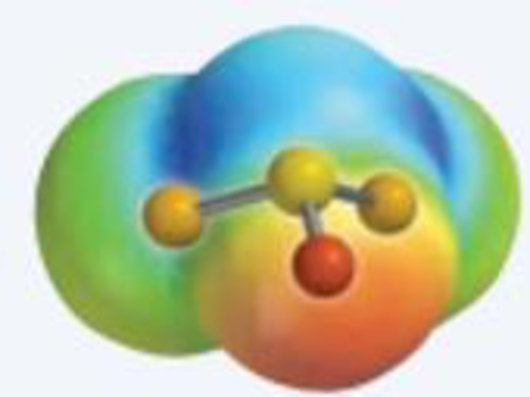Chapter 8.8, Problem 8.14CYU

Chapter
Section
Textbook Problem

The electrostatic potential surface for SOCl2 is pictured here. (a) Draw a Lewis electron dot picture for the molecule, and give the formal charge of each atom. (b) What is the molecular geometry of SOCl2? Is it polar?(a)

Interpretation Introduction

Interpretation: The Lewis electron dot structure for SOCl2 and the formal charge for each atom should be determined.

Concept Introduction:

• Lewis structures are diagrams that represent the chemical bonding of covalently bonded molecules and coordination compounds.
• It is also known as Lewis dot structures which represent the bonding between atoms of a molecule and the lone pairs of electrons that may exist in the molecule.
• The Lewis structure is based on the concept of the octet rule so that the electrons shared in each atom should have 8 electrons in its outer shell.

Lewis structure for any molecule is drawn by using the following steps,

First the skeletal structure for the given molecule is drawn then the total number of valence electrons for all atoms present in the molecule is determined

The next step is to subtract the electrons present in the total number of bonds present in the skeletal structure of the molecule with the total valence electrons such that considering each bond contains two electrons with it.

Finally, the electrons which got after subtractions have to be equally distributed such that each atom contains eight electrons in its valence shell.

Formal charge (FC): The charge assigned to an atom in a molecule, irrespective of relative electro negativity by thinking that electrons in all chemical bonds are shared equally among atoms.

Formal charge of an atom can be determined by the given formula.

Formalcharge(FC)=(numberofvalenceelectroninatom)[(numberofnon-bondingelectrons)+12(numberof bondingelectrons)]

Explanation

The Lewis structure of the SOCl2 molecules identified as follows,

The Lewis electron dot structure for given molecules are determined by first drawing the skeletal structure for the given molecules, then the total number of valence electrons for all atoms present in the molecules are determined.

The next step is to subtract the electrons present in the total number of bonds present in the skeletal structure of the molecule with the total valence electrons such that considering each bond contains two electrons with it.

Finally, the electrons which got after subtractions have to be equally distributed considering each atom contains eight electrons in its valence shell.

The formal charge of atoms present in SOCl2 are as follows,

Formalcharge(FC)=(numberofvalenceelectroninatom)[(numberofnon-bondingelectrons)+12(numberof bondingelectrons)]

The formal charge for atom in molecule is determined by plugging the number of valence bonded and non-bonded electrons around the respective atom in a molecule into the formula

(b)

Interpretation Introduction

Interpretation: The molecular geometry for the given molecule should be determined and it should be identified that whether it is polar or not.

Concept Introduction:

Polar molecule: The molecule with atoms bonded with different electronegativity. Dipole moment is used to measure the polarity of the molecule.

Molecular geometry: It is defined as unique three dimensional arrangements of atoms around the central metal present in the molecule which is determined by using spectroscopic techniques and also by using Lewis structure or the valence shell electron pair repulsion theory (VSEPR).

VSEPR Theory:

As the name itself indicates that the basis for this theory is the electron pair that is bonded electron present in either single or double bonds or lone pair electrons, present in the valence shell tends to repel each other which then the tends to be in position in order to minimize the repulsions. The steps involved in the theory in describing the geometry is as follows,

• The first step is to draw the correct Lewis structure for the molecule.
• Then, the electron domain around the central atom should be counted and the geometry that matches with that type of domain in VSEPR should be determined.
• Finally, the geometry is predicted by using the orientation of atoms.

The molecules with considering the domains of type AB2 will tend to have shape like linear or bent if the central atom have lone pair of electrons with it, type AB3 will have shape like trigonal planar, type AB4 will have shape like tetrahedral or square planar, type AB5 will have trigonal bipyramidal and AB6 will have shape like octahedral respectively.

Electron Domain: In VSEPR theory, both the lone pair and the bonded pair are together considered as electron domain regardless of the type of bond in which the bonded pair presents.

Electrostatic potential map: It is 3D representation of molecules which shows the distribution of charges present in the molecule. Generally red, orange, yellow, green and blue defines the charge potentials present in the molecule.

RED<ORANGE<YELLOW<GREEN<BLUE

The blue color indicates the most positive potential end and the red color defines the most negative potential end.

Still sussing out bartleby?

Check out a sample textbook solution.

See a sample solution

The Solution to Your Study Problems

Bartleby provides explanations to thousands of textbook problems written by our experts, many with advanced degrees!

Get Started

People most often choose foods for the nutrients they provide. T F

Nutrition: Concepts and Controversies - Standalone book (MindTap Course List)

What is the plasma membrane, and what are its main functions?

Biology: The Dynamic Science (MindTap Course List)

What are sister chromatids?

Human Heredity: Principles and Issues (MindTap Course List)